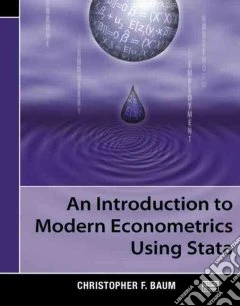ricerca
avanzata

# An Introduction to Modern Econometrics Using Stata

## Un libro in lingua di Baum Christopher F. edito da Statacorp Lp, 2006

• € 104,10
• Il prezzo è variabile in funzione del cambio della valuta d’origineIntegrating a contemporary approach to econometrics with the powerful computational tools offered by Stata, An Introduction to Modern Econometrics Using Stata focuses on the role of method-of-moments estimators, hypothesis testing, and specification analysis and provides practical examples that show how the theories are applied to real data sets using Stata.

As an expert in Stata, the author successfully guides readers from the basic elements of Stata to the core econometric topics. He first describes the fundamental components needed to effectively use Stata. The book then covers the multiple linear regression model, linear and nonlinear Wald tests, constrained least-squares estimation, Lagrange multiplier tests, and hypothesis testing of nonnested models. Subsequent chapters center on the consequences of failures of the linear regression model's assumptions. The book also examines indicator variables, interaction effects, weak instruments, underidentification, and generalized method-of-moments estimation. The final chapters introduce panel-data analysis and discrete- and limited-dependent variables and the two appendices discuss how to import data into Stata and Stata programming.

Presenting many of the econometric theories used in modern empirical research, this introduction illustrates how to apply these concepts using Stata. The book serves both as a supplementary text for undergraduate and graduate students and as a clear guide for economists and financial analysts.